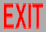# Reggeons meet partons

(CNYITP seminar, '05)

# Energy regions for hadrons

• Low: lattice, σ, instantons, nonrel. quarks
• Spectrum (high & low): Regge
• High, small angle (“soft”): Regge (sometimes pQCD)
• High, large angle (“hard”): pQCD

# Regge theory

• α(t) relates spectrum & high-E, small-θ
• Spin J = α(M2), Amplitude ∼ β(t)sα(t)
• “Small” coupling → α(t) = α’t + α0 linear
• Tree = poles → DHS (s↔t) duality (strings)
• Experiment: OK for -2 GeV2 ≤ t ≤ 6 GeV2;
α’ ≈ 1 GeV-2, α0 ≈ ½ (ρ,ω,...)
• Reggeons (mesons/baryons) easier than pomerons (glueballs): spectrum known, cuts don’t get in way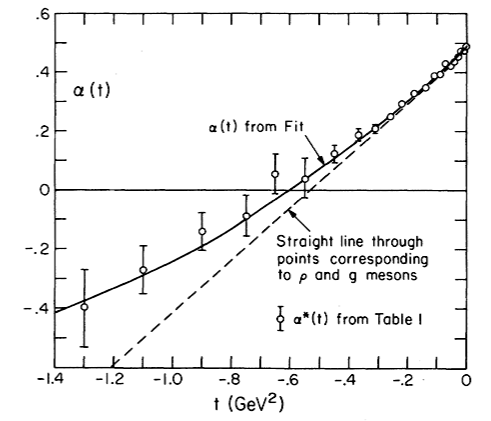#### Barnes et al. ‘76 (Fermilab data)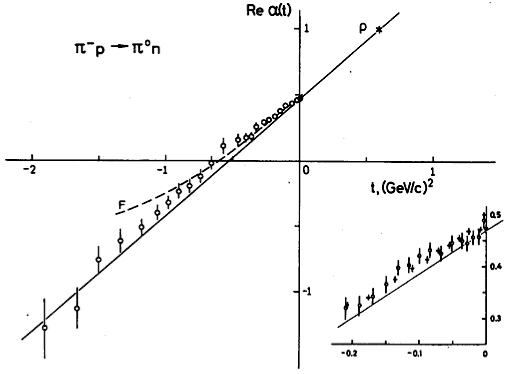### t < 0: πN charge exchange scattering (t-channel pole)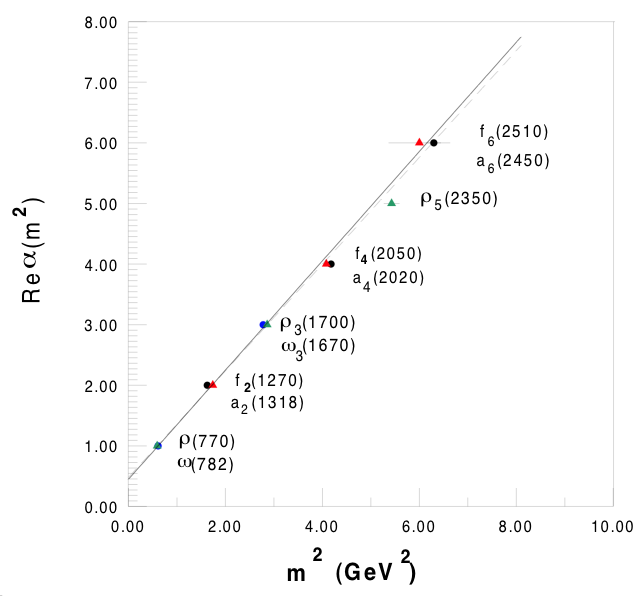# Partons in Reggeons?

• Strings → exponentials, not powers, for parton propagators (large transverse p)
• pQCD = nonperturbative strings?
• Sum cuts or “sister trajectories”?
• How to interpolate small θ ↔ large θ?

# AdS/CFT: Polchinski & Strassler

• AdS/CFT: 5th dimension = running tension
• P&S: α’ = cutoff on 5th D, introduces scale
• Continuum of trajectories, slope ≤ α’
• Regge & partons, but continuous spectrum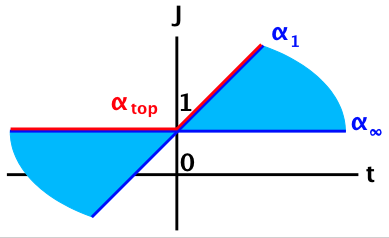# Our proposal

• over discrete tensions (not ∫ ) → discrete, integer-spaced spectrum
• Intercepts converge → partons
• Trees: leading order for both Reggeons & partons (cf. Gribov, Dokshitzer, Hoyer)

# Cuts & sisters

• Quantized tension (OK)
• Higher tension only at higher-pt. → useful only for Pomeron (total cross section)
• Intercepts don’t converge → no partons
• Cuts: αn(t) = α’t/n -bn +1
• Sisters: αn(t) = (α’t+α0)/n -½(n-1)

# Picture

• Many (straight) trajectories αn(t), but highest one (“top trajectory”) dominates
• For t > 0, α1(t) = α’t+α0 dominates → usual spectrum
• For -s ≪ t < 0, same or corrections from βn(t) → still Regge (as for P-S)
• For t ≪ 0, α(t) = const. dominates → usual parton behavior (as for P-S)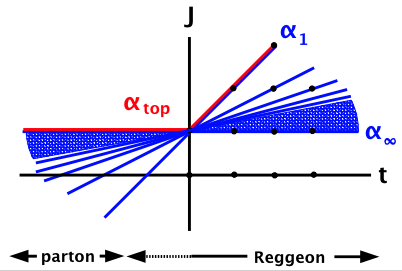# General set-up

• αn(t) has same poles as α1(t)α’M20 = an J + bn ,
an & bn integers → αn(t) = (α’t + α0 - bn)/an
• an & bn increasing, bn/an→ const. (n→∞)
• A = ∑wnAn , An = string amplitude, wn ≈ (c/n)an-c , c = ½ # quarks

# Simplest model

• αn(t) = α’(t-t0)/n + J0 , J0 integer
• For t ≥ t0, αtop(t) = α1(t) = α’(t-t0) +J0
• For t ≤ t0, αtop(t) = α(t) = J0
• With above wn, A ∼ sα1(t) also for t0 - t ≪ (c+1)/α’ln(α’s)
• Fixed θ, high E: A ∼ (α’s)2-c (also more generally)

# Interpretation

• Assume αtop(t) “turns” at t0=0 (as for P-S),
so α1(t) = α’t +α0, α(t) = α0
• α0 ≈ ½ for Reggeon, 1 for Pomeron
• Then α (large -t) represents “jet” (far off-shell hadron), with α0 = spin of parton carrying most of E (quark for Reggeon, gluon for Pomeron)
• So treat jet, not parton, as fundamental